Share

# Selina solutions for Concise Mathematics Class 10 ICSE chapter 18 - Tangents and Intersecting Chords [Latest edition]

Course
Textbook page

#### Chapters## Chapter 18: Tangents and Intersecting Chords

Exercise 18 (A)Exercise 18 (B)Exercise 18 (C)

#### Selina solutions for Concise Mathematics Class 10 ICSE Chapter 18 Tangents and Intersecting Chords Exercise Exercise 18 (A) [Pages 274 - 276]

Exercise 18 (A) | Q 1 | Page 274

The radius of a circle is 8 cm. calculate the length of a tangent draw to this circle from a point at a distance of 10 cm from its centre.

Exercise 18 (A) | Q 2 | Page 274

In the given figure, O is the center of the circle and AB is a tangent at B. If AB = 15 cm and AC = 7.5 cm, calculate the radius of the circle.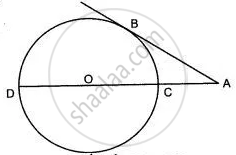Exercise 18 (A) | Q 3 | Page 274

Two circle touch each other externally at point P. Q is a point on the common tangent through P. Prove that the tangents QA and QB are equal.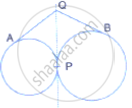Exercise 18 (A) | Q 4 | Page 274

Two circle touch each other internally. Show that the tangents drawn to the two circles from any point on the common tangent are equal in length.

Exercise 18 (A) | Q 5 | Page 274

Two circle of radii 5 cm and 3 cm are concentric. Calculate the length of a chord of the outer circle which touches the inner

Exercise 18 (A) | Q 6 | Page 274

Three circles touch each other externally. A triangle is formed when the centres of these circles are joined together. Find the radii of the circle, if the sides of the triangle formed are 6 cm, 8 cm and 9 cm

Exercise 18 (A) | Q 7 | Page 274

If the sides of a quadrilateral ABCD touch a circle, prove that:
AB + CD = BC + AD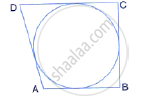Exercise 18 (A) | Q 8 | Page 275

If the sides of a parallelogram touch a circle in following figure , Prove that the parallelogram is a rhombus.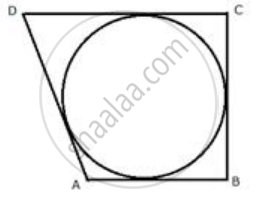Exercise 18 (A) | Q 9 | Page 275

From the given figure, prove that:
AP + BQ + CR = BP + CQ + AR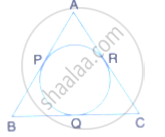Also show that:
AP + BQ + CR = 1/2× Perimeter of ΔABC.

Exercise 18 (A) | Q 10 | Page 275

In the following figure; If AB = AC then prove that BQ = CQ.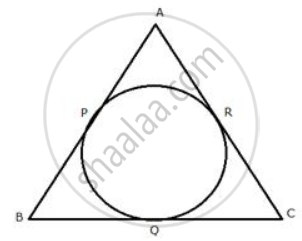Exercise 18 (A) | Q 11.1 | Page 275

Radii of two circles are 6. 3 cm and 3.6 cm. State the distance between their centres if:

they touch each other externally

Exercise 18 (A) | Q 11.2 | Page 275

Radii of two circles are 6.3 cm and 3.6 cm. State the distance between their centers if -

they touch each other internally.

Exercise 18 (A) | Q 12.1 | Page 275

From a point P outside a circle, with centre O, tangents PA and PB are drawn. Prove that:

∠AOP = ∠BOP

Exercise 18 (A) | Q 12.2 | Page 275

From a point P outside the circle, with centre O, tangents PA and PB are drawn. Prove that:

OP is the perpendicular bisector of chord AB.

Exercise 18 (A) | Q 13.1 | Page 275

In the given figure, two circles touch each other externally at point P. AB is the direct common tangent of these circles. Prove that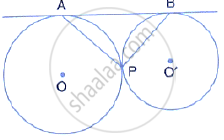tangent at point P bisects AB,

Exercise 18 (A) | Q 13.2 | Page 275

In the given figure, two circles touch each other externally at point P. AB is the direct common tangent of these circles. Prove that: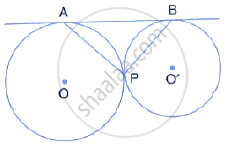(ii) angles APB = 90°

Exercise 18 (A) | Q 14 | Page 275

Tangents AP and AQ are drawn to a circle, with centre O, from an exterior point A. prove that: PAQ = 2 ∠OPQ

Exercise 18 (A) | Q 15 | Page 275

Two parallel tangents of a circle meet a third tangent at points P and Q. prove that PQ subtends a right angle at the centre.

Exercise 18 (A) | Q 16 | Page 275

ABC is a right angles triangle with AB = 12 cm and AC = 13 cm. A circle, with centre O, has been inscribed inside the triangle.
Calculate the value of x, the radius of the inscribed circle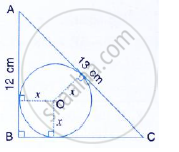Exercise 18 (A) | Q 17.1 | Page 275

In a triangle ABC, the incircle (centre O) touches BC, CA and AB at points P, Q and R respectively. Calculate : (i) ∠QOR

Exercise 18 (A) | Q 17.2 | Page 275

In a triangle ABC, the incircle (centre O) touches BC, CA and AB at points P, Q and R respectively. Calculate:

i)∠QPR .

Exercise 18 (A) | Q 18.1 | Page 275

In the following figure, PQ and PR are tangents to the circle, with centre O. If ∠QPR = 60°, calculate:

∠QOR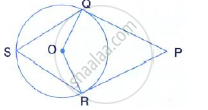Exercise 18 (A) | Q 18.2 | Page 275

In the following figure, PQ and PR are tangents to the circle, with centre O. If ∠ QPR = 60° , calculate:

∠ OQR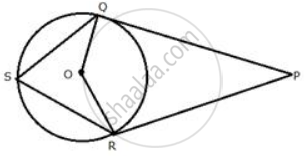Exercise 18 (A) | Q 18.3 | Page 275

In the following figure, PQ and PR are tangents to the circle, with centre O. If ∠ QPR = 60° , calculate:

∠ QSR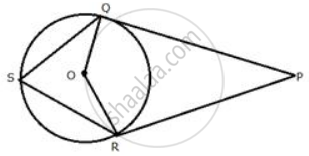Exercise 18 (A) | Q 19 | Page 275

In the given figure, AB is the diameter of the circle, with centre O, and AT is the tangent. Calculate the numerical value of x.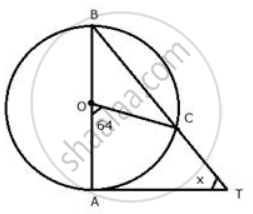Exercise 18 (A) | Q 20 | Page 275

In quadrilateral ABCD; angles D = 90°, BC = 38 cm and DC = 25 cm. A circle is inscribed in this quadrilateral which touches AB at point Q such that QB = 27 cm, Find the radius of the circle.

Exercise 18 (A) | Q 21 | Page 275

In the given figure, PT touches the circle with centre O at point R. Diameter SQ is produced to meet the tangent TR at P.
Given ∠SPR = x° and ∠QRP = y°;
Prove that:
(i) ∠ORS = y°
(ii) Write an expression connecting x and y.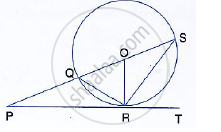Exercise 18 (A) | Q 22.1 | Page 276

PT is a tangent to the circle at T. if ∠ABC = 70° and ∠ACB = 50°; Calculate:

∠ CBT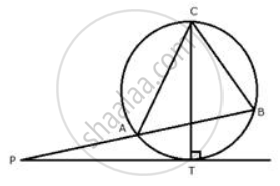Exercise 18 (A) | Q 22.2 | Page 276

PT is a tangent to the circle at T. If ∠ ABC = 70° and ∠ ACB = 50° ; calculate : ∠ APT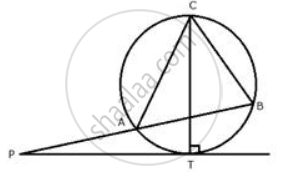Exercise 18 (A) | Q 22.3 | Page 276

PT is a tangent to the circle at T. If ∠ ABC = 70° and ∠ ACB = 50° ; calculate : ∠ APT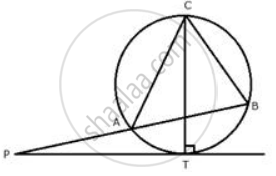Exercise 18 (A) | Q 23 | Page 276

In the given figure, O is the centre of the circumcircle ABC. Tangents at A and C intersect at P. Given angle AOB = 140° and angle APC = 80°; find the angle BAC.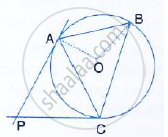Exercise 18 (A) | Q 24 | Page 276

In the given figure, PQ is a tangent to the circle at A. AB and AD are bisectors of ∠CAQ and ∠PAC. If ∠BAQ = 30°, prove that : BD is diameter of the circle.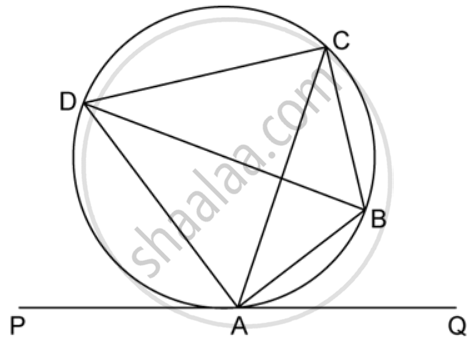#### Selina solutions for Concise Mathematics Class 10 ICSE Chapter 18 Tangents and Intersecting Chords Exercise Exercise 18 (B) [Pages 283 - 284]

Exercise 18 (B) | Q 1.1 | Page 283

i) In the given figure 3 × CP = PD = 9cm and AP = 4.5 cm Find BP.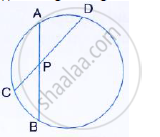Exercise 18 (B) | Q 1.2 | Page 283

(ii) In the given figure, 5 × PA = 3 × AB = 30 cm and PC = 4cm. find CD.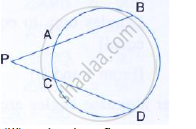Exercise 18 (B) | Q 1.3 | Page 283

(iii) In the given figure tangent PT = 12.5 cm and PA = 10 cm; find AB.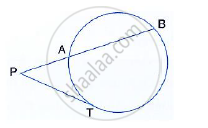Exercise 18 (B) | Q 2.1 | Page 283

In the given figure, diameter AB and chord CD of a circle meet at P. PT is a tangent to the circle at T. CD = 7.8 cm, PD = 5 cm, PB = 4 cm. Find AB.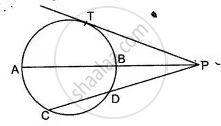Exercise 18 (B) | Q 2.2 | Page 283

In the given figure, diameter AB and chord CD of a circle meet at P. PT is a tangent to the circle at T. CD = 7.8 cm, PD = 5 cm, PB = 4 cm. Find the length of tangent PT.Exercise 18 (B) | Q 3.1 | Page 283

In the following figure, PQ is the tangent to the circle at A, DB is the diameter and O is the centre of the circle. If ∠ADB = 30° and ∠CBD = 60°, Calculate: ∠QAB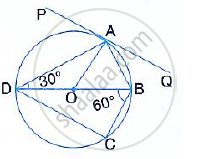Exercise 18 (B) | Q 3.2 | Page 283

In the following figure, PQ is the tangent to the circle at A, DB is a diameter and O is the centre of the circle. If ∠ ADB = 30° and ∠ CBD = 60° ; calculate ∠ PAD.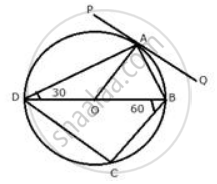Exercise 18 (B) | Q 3.3 | Page 283

In the following figure, PQ is the tangent to the circle at A, DB is a diameter and O is the centre of the circle. If ∠ ADB = 30° and ∠ CBD = 60° ; calculate : ∠CDB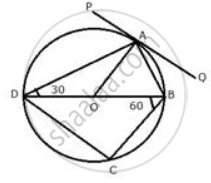Exercise 18 (B) | Q 4 | Page 283

If PQ is a tangent to the circle at R; calculate:
(i) ∠PRS

(ii) ∠ROT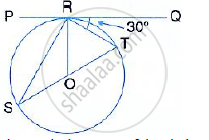Given :  O is the centre of the circle and angle TRQ = 30°

Exercise 18 (B) | Q 5 | Page 283

AB is the diameter and AC is a chord of a circle with centre O such that angle BAC = 30°. The tangent to the circle at C intersects AB produced in D. show that BC = BD.

Exercise 18 (B) | Q 6 | Page 283

Tangent at P to the circumcircle of triangle PQR is drawn. If the tangent is parallel to side, QR show that ΔPQR is isosceles.

Exercise 18 (B) | Q 7 | Page 284

Two circle with centres O and O ' are drawn to intersect each other at points A and B.
Centre O of one circle lies on the circumference of the other circle and CD is drawn tangent to the circle with centre O ' at A. prove that OA bisects angle BAC.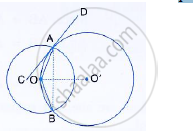Exercise 18 (B) | Q 8 | Page 284

Two circles touch each other internally at a point P. A chord AB of the bigger circle intersects the other circle in C and D. Prove that ∠CPA = ∠DPB.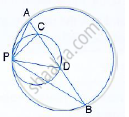Exercise 18 (B) | Q 9 | Page 284

In a cyclic quadrilateral ABCD, the diagonal AC bisects the angle BCD. Prove that the diagonal BD is parallel to the tangent to the circle at point A.

Exercise 18 (B) | Q 10.1 | Page 284

In the figure, ABCD is a cyclic quadrilateral with BC = CD. TC is tangent to the circle at point C and DC is produced to point G. If ∠BCG = 108° and O is the centre of the circle, find : Angle BCT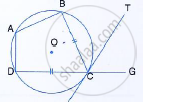Exercise 18 (B) | Q 10.2 | Page 284

In the figure, ABCD is a cyclic quadrilateral with BC = CD. TC is tangent to the circle at point C and DC is produced to point G. If angle BCG=108°  and O is the centre of the circle, find: angle DOC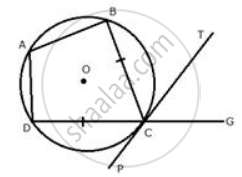Exercise 18 (B) | Q 11 | Page 284

Two circles intersect each other at points A and B. A straight line PAQ cuts the circles at P and Q. If the tangents at P and Q intersect at point T; show that the points P, B, Q and T are concyclic

Exercise 18 (B) | Q 12 | Page 284

In the figure; PA is a tangent to the circle, PBC is secant and AD bisects angle BAC. Show that triangle PAD is an isosceles triangle. Also, show that:

∠CAD =1/2(∠PBA-∠PAB)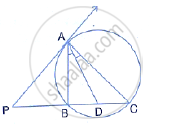Exercise 18 (B) | Q 13 | Page 284

Two circles intersect each other at points A and B. their common tangent touches the circles at points P and Q as shown in the figure. Show that the angles PAQ and PBQ are supplementary.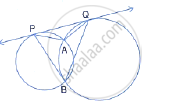Exercise 18 (B) | Q 14.1 | Page 284

In the figure, chords AE and BC intersect each other at point D.
If ∠ CDE = 90°, AB = 5 cm, BD = 4 cm and CD = 9 cm
Find DE.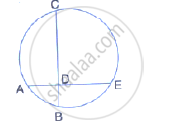Exercise 18 (B) | Q 14.2 | Page 284

In the figure, chords AE and BC intersect each other at point D.

If AD = BD, Show that AE = BC.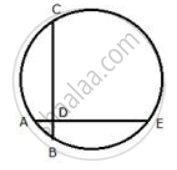Exercise 18 (B) | Q 15 | Page 284

Circles with centres P and Q intersect at points A and B as shown in the figure. CBD is a segment and EBM is tangent to the circle with centre Q, at point B. If the circle are congruent; show that
CE = BD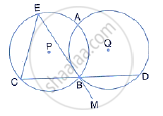Exercise 18 (B) | Q 16 | Page 284

In the adjoining figure, O is the centre of the circle and AB is a tangent to it at point B. ∠BDC = 65° Find ∠BAO.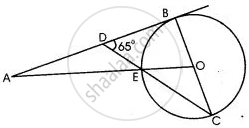#### Selina solutions for Concise Mathematics Class 10 ICSE Chapter 18 Tangents and Intersecting Chords Exercise Exercise 18 (C) [Pages 285 - 288]

Exercise 18 (C) | Q 1 | Page 285

Prove that, of any two chords of a circle, the greater chord is nearer to the centre.

Exercise 18 (C) | Q 2.1 | Page 285

OABC is a rhombus whose three vertices A, B and C lie on a circle with centre O.
(i) If the radius of the circle is 10 cm, find the area of the rhombus

Exercise 18 (C) | Q 2.2 | Page 285

OABC is a rhombus whose three vertices A, B and C lie on a circle with centre O.

(ii) If the area of the rhombus is 32 3 cm2 find the radius of the circle.

Exercise 18 (C) | Q 3 | Page 285

Two circle with centres A and B, and radii 5 cm and 3 cm, touch each other internally. If the perpendicular bisector of the segment AB meets the bigger circle in P and Q; find the length of PQ.

Exercise 18 (C) | Q 4 | Page 285

Two chords AB and AC of a circle are equal. Prove that the centre of the circle lies on the bisector of angle BAC.

Exercise 18 (C) | Q 5 | Page 285

The diameter and a chord of a circle have a common end-point. If the length of the diameter is 20 cm and the length of the chord is 12 cm, how far is the chord from the centre of the circle?

Exercise 18 (C) | Q 6 | Page 285

ABCD is a cyclic quadrilateral in which BC is parallel to AD, angle ADC = 110° and angle BAC = 50°. Find angle DAC and angle DCA.

Exercise 18 (C) | Q 7 | Page 285

In the given figure, C and D are points on the semi-circle described on AB as diameter. Given angle BAD = 70° and angle DBC = 30°, calculate angle BDC.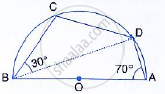Exercise 18 (C) | Q 8 | Page 285

In cyclic quadrilateral ABCD, ∠A = 3 ∠C and ∠D = 5 ∠B. Find the measure of each angle of the quadrilateral.

Exercise 18 (C) | Q 9 | Page 285

Show that the circle drawn on any one of the equal sides of an isosceles triangle as diameter bisects the base.

Exercise 18 (C) | Q 10 | Page 285

Bisectors of vertex angles A, B, and C of a triangle ABC intersect its circumcircle at the points D, E and F respectively. Prove that angle EDF  = 90° - 1/2 ∠A

Exercise 18 (C) | Q 11 | Page 285

In the figure, AB is the chord of a circle with centre O and DOC is a line segment such that BC = DO. If ∠C = 20°, find angle AOD.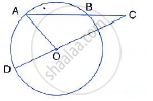Exercise 18 (C) | Q 12 | Page 285

Prove that the perimeter of a right triangle is equal to the sum of the diameter of its incircle and twice the diameter of its circumcircle.

Exercise 18 (C) | Q 13 | Page 285

P is the mid – point of an arc APB of a circle. Prove that the tangent drawn at P will be parallel  to the chord AB.

Exercise 18 (C) | Q 14 | Page 285

In the given figure, MN is the common chord of two intersecting circles and AB is their common tangent.Prove that the line NM produced bisects AB at P.

Exercise 18 (C) | Q 15 | Page 285

In the given figure, ABCD is a cyclic quadrilateral, PQ is tangent to the circle at point C and BD is its diameter. If ∠DCQ = 40° and ∠ABD = 60°, find;

(i) ∠DBC (ii) ∠BCP (iii) ∠ADB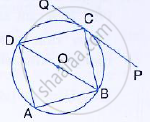Exercise 18 (C) | Q 16 | Page 285

The given figure shows a circle with centre O and BCD is tangent to it at C. Show that: ∠ACD + ∠BAC = 90°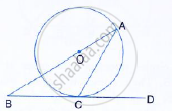Exercise 18 (C) | Q 17.1 | Page 286

ABC is a right triangle with angle B = 90°, A circle with BC as diameter meets hypotenuse AC at point D. prove that:
AC × AD = "AB"^2

Exercise 18 (C) | Q 17.2 | Page 286

ABC is a right triangle with angle B = 90º. A circle with BC as diameter meets by hypotenuse AC at point D.

Prove that - BD2 = AD x DC.

Exercise 18 (C) | Q 18 | Page 286

In the given figure, AC = AE Show that:
(i) CP = EP
(ii) BP = DP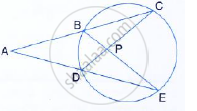Exercise 18 (C) | Q 19.1 | Page 286

ABCDE is a cyclic pentagon with centre of its circumcircle at point O such that AB = BC = CD and angle ABC = 120°

Calculate :  ∠BEC

Exercise 18 (C) | Q 19.2 | Page 286

ABCDE is a cyclic pentagon with centre of its circumcircle at point O such that AB = BC = CD and angle ABC=120°.

Calculate: ∠ BED.

Exercise 18 (C) | Q 20.1 | Page 286

In the given figure, O is the centre of the circle. Tangents at A and B meet at C. If ∠ACO = 30°, find: ∠BCO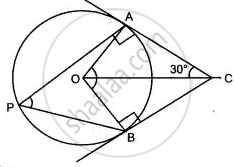Exercise 18 (C) | Q 20.2 | Page 286

In the given figure, O is the centre of the circle. Tangents at A and B meet at C. If angle ACO = 30°, find: angle AOB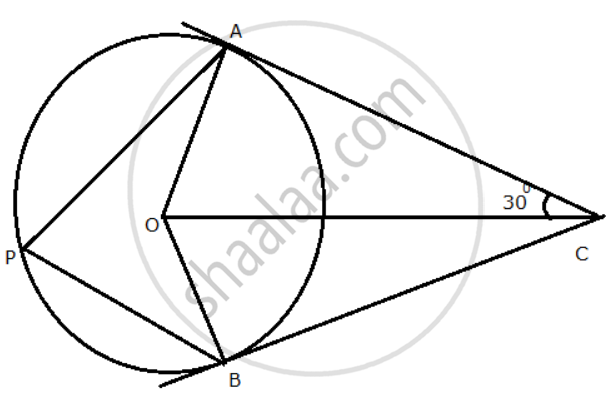Exercise 18 (C) | Q 20.3 | Page 286

In the given figure, O is the centre of the circle. Tangents at A and B meet at C. If angle ACO = 30°, find: angle APBExercise 18 (C) | Q 21 | Page 286

ABC is a triangle with AB = 10 cm, BC = 8 cm and AC = 6 cm (not drawn to scale). Three circle are drawn touching each other with the vertices as their centres. Find the radii of the three circles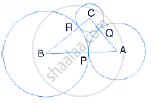Exercise 18 (C) | Q 22.1 | Page 286

In a square ABCD, its diagonals AC and BD intersect each other at point O. the bisector of angle DAO meets BD at point M and the bisector of angle ABD meets AC at N and AM at L. Show that:
∠ ONL + ∠ OML = 180

Exercise 18 (C) | Q 22.2 | Page 286

In a square ABCD, its diagonal AC and BD intersect each other at point O. The bisector of angle DAO meets BD at point M and bisector of angle ABD meets AC at N and AM at L. Show that -  ∠ BAM = ∠ BMA

Exercise 18 (C) | Q 22.3 | Page 286

In a square ABCD, its diagonal AC and BD intersect each other at point O. The bisector of angle DAO meets BD at point M and bisector of angle ABD meets AC at N and AM at L. Show that - ALOB is a cyclic quadrilateral.

Exercise 18 (C) | Q 23 | Page 286

The given figure shows a semi-circle with centre O and diameter PQ. If PA = AB and ∠BCQ =140°; Find measures of angles PAB and AQB. Also, show that AO is parallel to BQ.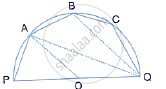Exercise 18 (C) | Q 24.1 | Page 286

The given figure shows a circle with centre O such that chord RS is parallel to chord QT, angle PRT = 20° and angle POQ = 100°. Calculate:

(i) angle QTR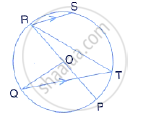Exercise 18 (C) | Q 24.2 | Page 286

The given figure shows a circle with centre O such that chord RS is parallel to chord QT, angle PRT = 20° and angle POQ = 100°. Calculate:

(ii) angle QRP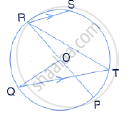Exercise 18 (C) | Q 24.3 | Page 286

The given figure shows a circle with centre O such that chord RS is parallel to chord QT, angle PRT = 20° and angle POQ = 100°. Calculate:

(iii) angle QRS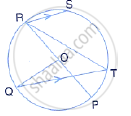Exercise 18 (C) | Q 24.4 | Page 286

The given figure shows a circle with centre O such that chord RS is parallel to chord QT, angle PRT = 20° and angle POQ = 100°. Calculate:

(iv) angle STR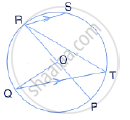Exercise 18 (C) | Q 25 | Page 286

In the given figure, PAT is tangent to the circle with centre O at point A on its circumference and is parallel to chord BC. If CDQ is a line segment , show that: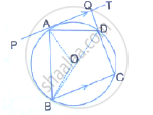Exercise 18 (C) | Q 26 | Page 286
AB is a line segment and M is its mid – point three semi circles are drawn with AM, MB and AB as diameter on the same side of the line AB. A circle with radius r unit is drawn so  that it  touches all the three semi circles show that : AB = 6 × r
Exercise 18 (C) | Q 27 | Page 286

TA and TB are tangents to a circle with centre O from an external point T. OT intersects the circle at point P. Prove that AP bisects the angle TAB.

Exercise 18 (C) | Q 28 | Page 286

Two circles intersect in points P and Q. A secant passing through P intersects the circles in Aand B respectively. Tangents to the circles at A and B intersect at T. Prove that A, Q, B and T lie on a circle.

Exercise 18 (C) | Q 29 | Page 286

Prove that any four vertices of a regular pentagon are concylic (lie on the same circle)

Exercise 18 (C) | Q 30 | Page 286

Chords AB and CD of a circle when extended meet at point X. Given AB = 4 cm, BX = 6 cm and XD = 5 cm, calculate the length of CD.

Exercise 18 (C) | Q 31 | Page 287

In the given figure, find TP if AT = 16 cm and AB = 12 cm.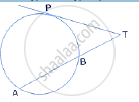Exercise 18 (C) | Q 32 | Page 287

In the following figure, a circle is inscribed in the quadrilateral ABCD.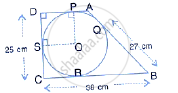If BC = 38 cm, QB = 27 cm, DC = 25 cm and that AD is perpendicular to DC, find the radius of the circle.

Exercise 18 (C) | Q 33 | Page 287

In the given figure, XY is the diameter of the circle and PQ is a tangent to the circle at Y.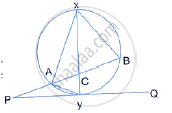If ∠AXB = 50° and ∠ABX = 70° and ∠BAY and ∠APY

Exercise 18 (C) | Q 34 | Page 287

In the given figure, QAP is the tangent at point A and PBD is a straight line.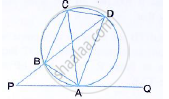If ∠ACB = 36° and ∠APB = 42°, find:
(i) ∠BAP (ii) ∠ABD (iii) ∠QAD (iv) ∠BCD

Exercise 18 (C) | Q 35.1 | Page 287

In the given figure, AB is the diameter. The tangent at C meets AB produced at Q.
If ∠CAB = 34°, Find : ∠CBA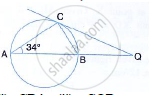Exercise 18 (C) | Q 35.2 | Page 287

In the given figure, AB is the diameter. The tangent at C meets AB produced at Q.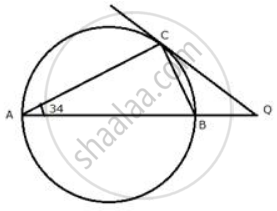If ∠ CAB = 34° , find : ∠ CQB

Exercise 18 (C) | Q 36 | Page 287

In the given figure, O is the centre of the circle. The tangents at B and D intersect each other at point P. If AB is parallel to CD and ∠ABC = 55°, find: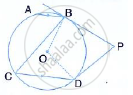(i) ∠BOD (ii) ∠BPD

Exercise 18 (C) | Q 37 | Page 287

In two concentric circles, prove that all chords of the outer circle, which touch the inner circle, are of equal length.

Exercise 18 (C) | Q 38 | Page 287

In the following figure, PQ = QR, ∠RQP  = 68° , PC and CQ are tangents to the circle with centre O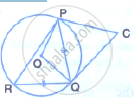(i) ∠QOP
(ii) ∠QCP

Exercise 18 (C) | Q 39 | Page 287

In the figure, given below, AC is a transverse common tangent to two circles with centres P and Q and of radii 6 cm and 3 cm respectively.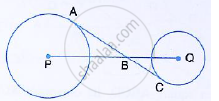Given that AB = 8 cm, calculate PQ.

Exercise 18 (C) | Q 40 | Page 287

In the figure, given below, O is the centre of the circumcircle of triangle XYZ.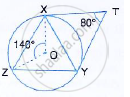Tangents at X and Y intersect at point T. Given ∠XTY = 80°, and ∠XOZ = 140°, calculate the  value of ∠ZXY.

Exercise 18 (C) | Q 41 | Page 288

In the given figure, AE and BC intersect each other as point D.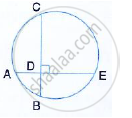If ∠CDE = 90°, AB = 5cm, BD = 4cm and CD = 9 cm find AE.

Exercise 18 (C) | Q 42 | Page 288

In the given circle with center o, ∠ABC=100°, ∠ACD=40° and CT is tangent to the circle at C. find ∠ADC and ∠DCT.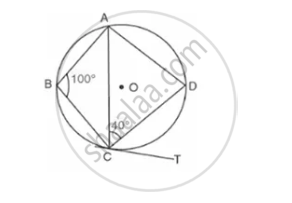Exercise 18 (C) | Q 43 | Page 288

In the figure given below, O is the center of the circle of the circle and SP is a tangent. if ∠SRT=65°, find the value of x, y and Z.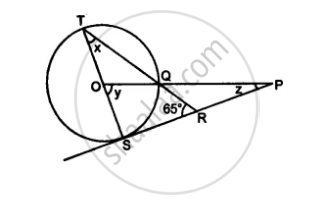## Chapter 18: Tangents and Intersecting Chords

Exercise 18 (A)Exercise 18 (B)Exercise 18 (C)## Selina solutions for Concise Mathematics Class 10 ICSE chapter 18 - Tangents and Intersecting Chords

Selina solutions for Concise Mathematics Class 10 ICSE chapter 18 (Tangents and Intersecting Chords) include all questions with solution and detail explanation. This will clear students doubts about any question and improve application skills while preparing for board exams. The detailed, step-by-step solutions will help you understand the concepts better and clear your confusions, if any. Shaalaa.com has the CISCE Concise Mathematics Class 10 ICSE solutions in a manner that help students grasp basic concepts better and faster.

Further, we at Shaalaa.com provide such solutions so that students can prepare for written exams. Selina textbook solutions can be a core help for self-study and acts as a perfect self-help guidance for students.

Concepts covered in Concise Mathematics Class 10 ICSE chapter 18 Tangents and Intersecting Chords are Tangent to a Circle, Number of Tangents from a Point on a Circle, Chord Properties - a Straight Line Drawn from the Center of a Circle to Bisect a Chord Which is Not a Diameter is at Right Angles to the Chord, Chord Properties - the Perpendicular to a Chord from the Center Bisects the Chord (Without Proof), Chord Properties - Equal Chords Are Equidistant from the Center, Chord Properties - Chords Equidistant from the Center Are Equal (Without Proof), Chord Properties - There is One and Only One Circle that Passes Through Three Given Points Not in a Straight Line, Arc and Chord Properties - the Angle that an Arc of a Circle Subtends at the Center is Double that Which It Subtends at Any Point on the Remaining Part of the Circle, Arc and Chord Properties - Angles in the Same Segment of a Circle Are Equal (Without Proof), Arc and Chord Properties - Angle in a Semi-circle is a Right Angle, Arc and Chord Properties - If Two Arcs Subtend Equal Angles at the Center, They Are Equal, and Its Converse, Arc and Chord Properties - If Two Chords Are Equal, They Cut off Equal Arcs, and Its Converse (Without Proof), Arc and Chord Properties - If Two Chords Intersect Internally Or Externally Then the Product of the Lengths of the Segments Are Equal, Cyclic Properties, Concept of Circles, Areas of Sector and Segment of a Circle, Tangent Properties - If a Line Touches a Circle and from the Point of Contact, a Chord is Drawn, the Angles Between the Tangent and the Chord Are Respectively Equal to the Angles in the Corresponding Alternate Segments, Tangent Properties - If a Chord and a Tangent Intersect Externally, Then the Product of the Lengths of Segments of the Chord is Equal to the Square of the Length of the Tangent from the Point of Contact to the Point of Intersection, Tangent Properties - If Two Circles Touch, the Point of Contact Lies on the Straight Line Joining Their Centers.

Using Selina Class 10 solutions Tangents and Intersecting Chords exercise by students are an easy way to prepare for the exams, as they involve solutions arranged chapter-wise also page wise. The questions involved in Selina Solutions are important questions that can be asked in the final exam. Maximum students of CISCE Class 10 prefer Selina Textbook Solutions to score more in exam.

Get the free view of chapter 18 Tangents and Intersecting Chords Class 10 extra questions for Concise Mathematics Class 10 ICSE and can use Shaalaa.com to keep it handy for your exam preparation